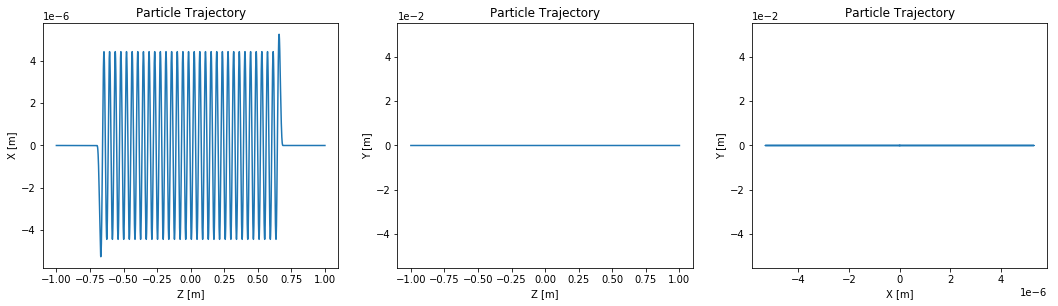# Example 007: Importing Trajectories¶

It is possible to import an externally calculated trajectory to oscars.sr. The trajectory may be input in the OSCARS trajectory format directly from python or it can be input from a text file with user specified input format. For inputting from a file the minimum required is time and position in at least 1 spatial dimension.

Defining a beam is required before importing so that OSCARS can associate the trajectory with a beam (charge required for calculations). Note that ctstart and ctstop are not needed. Calculations will be based on the input trajectry time.

This example will serve as a guide for inputting trajectories of various types.

If only the position is given the first and second derivatives are calculated from the derivative coefficients of a cubic spline. Higher order derivatives are less accurate and it is up to the user to dertermine if this is sufficient for the purpose.

## Standard setup and importing¶

In :
# Inline plots
%matplotlib inline

# Import oscars modules and plotting tools
import oscars.sr
from oscars.plots_mpl import *

# Other python imports
import numpy as np

OSCARS v2.1.8 - Open Source Code for Advanced Radiation Simulation
Brookhaven National Laboratory, Upton NY, USA
http://oscars.bnl.gov
oscars@bnl.gov

In :
# Create an oscars.sr object for calculations (use the GPU if you can!)

# Define a beam before we import any trajectories
# For imported trajectories ctstartstop is not needed, but is if you use internal propogation
osr.set_particle_beam(type='electron', current=0.5)


## Import Trajectory as a Python List¶

The trajectory must be in the OSCARS trajectory format:

• [[time, [x, y, z], [bx, by, bz], [bpx, bpy, bpz]], ...] where b refers to the relativistic beta (velicoty divided by speed of light) and bp is beta' or acceleration divided by the speed of light.
In :
# Importation of a python list trajectory.  This is just a straight trajectory.
beta = 0.9999999854933347
my_trajectory = [[t, [0, 0, t*beta*osr.c()], [0, 0, beta], [0, 0, 0]] for t in np.linspace(0, 1e-7, 100)]
osr.set_trajectory(trajectory=my_trajectory)

# Plot the trajectory
plot_trajectory_position(osr.get_trajectory())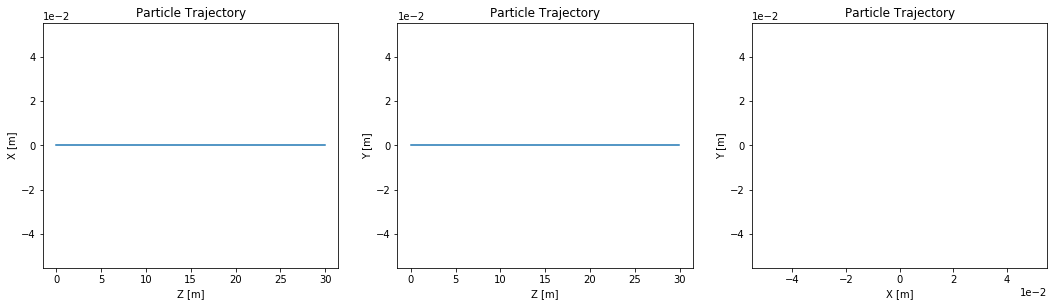In :
# It is possible to let OSCARS calculate the beta and beta' terms, just leave them out
# of the trajectory
beta = 0.9999999854933347
my_trajectory = [[t, [1e-6 * np.sin(2.*osr.pi()*t*beta*osr.c()/0.050), 0, t*beta*osr.c()]] for t in np.linspace(0, 1e-8, 10000)]
osr.set_trajectory(trajectory=my_trajectory)

# Plot the trajectory
plot_trajectory_position(osr.get_trajectory())
plot_trajectory_velocity(osr.get_trajectory())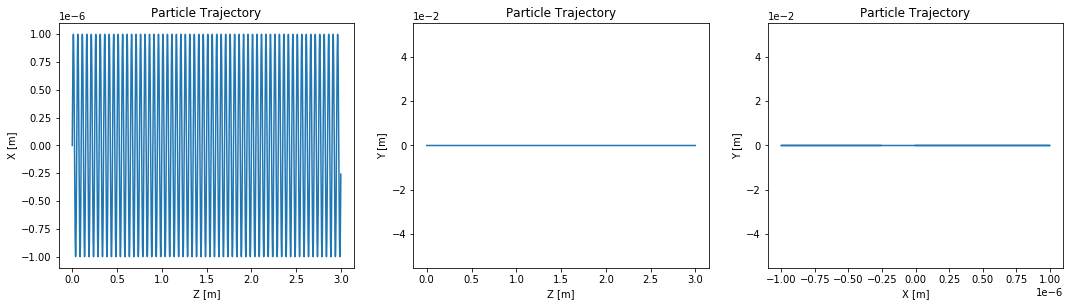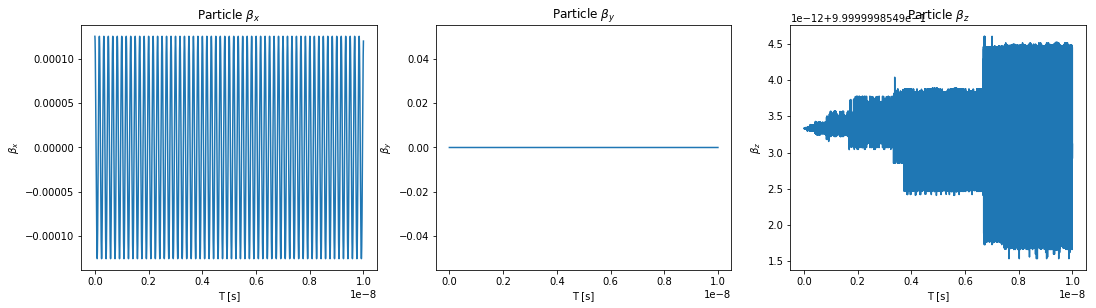In :
# As an example we calculate the power density
pd = osr.calculate_power_density_rectangle(plane='XY', width=[0.01, 0.01], npoints=[51, 51], translation=[0, 0, 30])
plot_power_density(pd)
print('Total Power:', osr.calculate_total_power(), '[W]')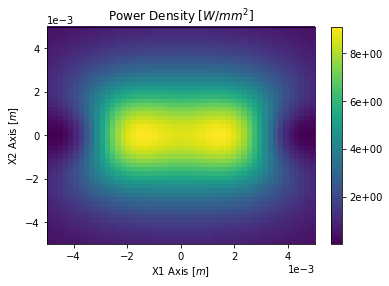Total Power: 409.6881272139957 [W]


## Importing Data: Text File¶

Data can be imported from a text file in column format. These must be spece delimited columns. You can specify the file format with the 'iformat' parameter. The default format for this is 'T X Y Z BX BY BZ BPX BPY BPZ'. At a minimum T and one of (X, Y, Z) must be specified. '*' in iformat will ignore a column.

Below we first create some trajectory in the default format, then read it in.

In :
# Create an EPU trajectory.  Here we need to define a beam with ctstartstop
osr.set_particle_beam(beam='NSLSII', x0=[0, 0, -1], ctstartstop=[0, 2])
osr.clear_bfields()
osr.add_bfield_undulator(bfield=[0, 1, 0], period=[0, 0, 0.042], nperiods=31, phase=-0.25*osr.pi())
osr.add_bfield_undulator(bfield=[1, 0, 0], period=[0, 0, 0.042], nperiods=31, phase=+0.25*osr.pi())

# Calculate trajectory and export it to a file
t = osr.calculate_trajectory(ofile='Example_007_Trajectory1.txt')
plot_trajectory_position(t)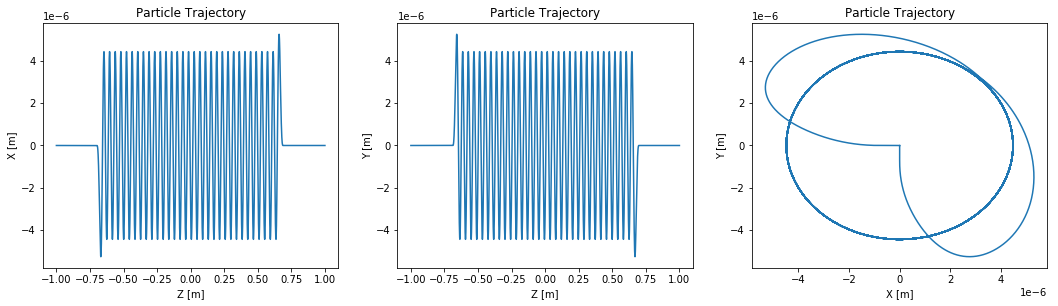Now that the trajectory has been created we can read it in using the 'ifile' argument

In :
# Read trajectory in default format
osr.set_trajectory(ifile='Example_007_Trajectory1.txt')

# Get and plot the trajectory
t = osr.get_trajectory()
plot_trajectory_position(t)
plot_trajectory_velocity(t)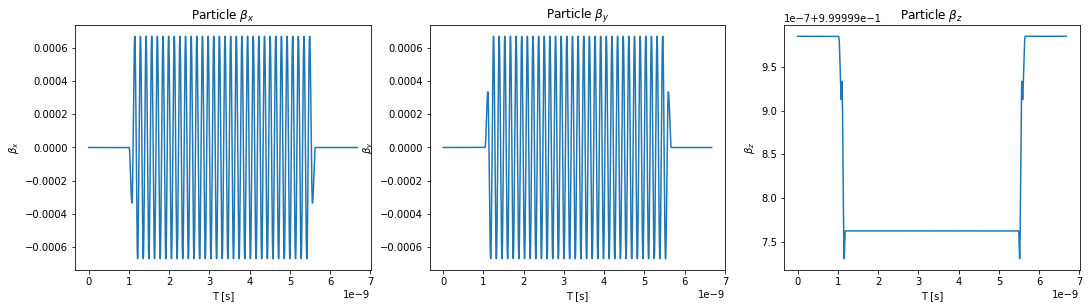In :
# As an example we can now calculate the power density
pd = osr.calculate_power_density_rectangle(plane='XY', width=[0.1, 0.1], npoints=[51, 51], translation=[0, 0, 30])
plot_power_density(pd)
print('Total Power:', osr.calculate_total_power(), '[W]')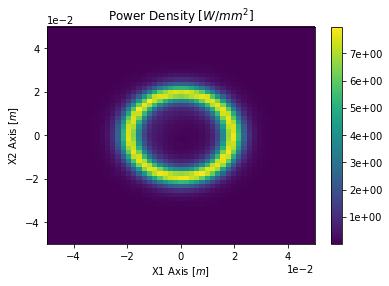Total Power: 7589.113887984705 [W]


If we only ask for the time and space components, OSCARS will calculate the beta and beta' terms

In :
# Read trajectory in default format
osr.set_trajectory(ifile='Example_007_Trajectory1.txt', iformat='T X Y Z * * * * * *')

# Get and plot the trajectory
t = osr.get_trajectory()
plot_trajectory_position(t)
plot_trajectory_velocity(t)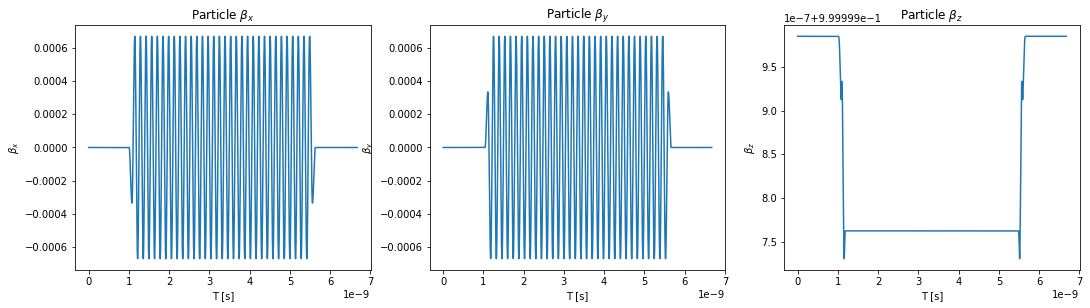In :
# As an example we can now calculate the power density
pd = osr.calculate_power_density_rectangle(plane='XY', width=[0.1, 0.1], npoints=[51, 51], translation=[0, 0, 30])
plot_power_density(pd)
print('Total Power:', osr.calculate_total_power(), '[W]')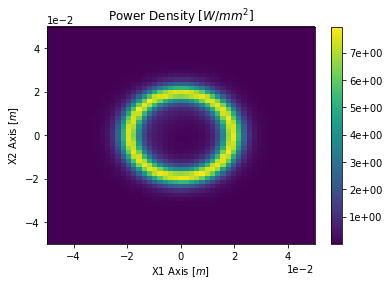Total Power: 7563.337199206862 [W]


## Ignore Columns¶

One can ignore columns in the input format using '*'. This is given as an example of how to ignore a column of data only and it is not recommended to ignore non-zero trajectory components since they are used in SR calculations. Note that in the example below beta and beta' will be technically incorrect.

This feature is meant only for ignoring extra data columns. Do not ignore trajectory data.

In :
# Read trajectory in default format
osr.set_trajectory(ifile='Example_007_Trajectory1.txt', iformat='T X * Z')

# Get and plot the trajectory
t = osr.get_trajectory()
plot_trajectory_position(t)
plot_trajectory_velocity(t)